# HSSlive: Plus One & Plus Two Notes & Solutions for Kerala State Board

## BSEB Class 9 Maths Chapter 12 Heron’s Formula Ex 12.1 Textbook Solutions PDF: Download Bihar Board STD 9th Maths Chapter 12 Heron’s Formula Ex 12.1 Book AnswersBSEB Class 9 Maths Chapter 12 Heron’s Formula Ex 12.1 Textbook Solutions PDF: Download Bihar Board STD 9th Maths Chapter 12 Heron’s Formula Ex 12.1 Book Answers

BSEB Class 9th Maths Chapter 12 Heron’s Formula Ex 12.1 Textbooks Solutions and answers for students are now available in pdf format. Bihar Board Class 9th Maths Chapter 12 Heron’s Formula Ex 12.1 Book answers and solutions are one of the most important study materials for any student. The Bihar Board Class 9th Maths Chapter 12 Heron’s Formula Ex 12.1 books are published by the Bihar Board Publishers. These Bihar Board Class 9th Maths Chapter 12 Heron’s Formula Ex 12.1 textbooks are prepared by a group of expert faculty members. Students can download these BSEB STD 9th Maths Chapter 12 Heron’s Formula Ex 12.1 book solutions pdf online from this page.

## Bihar Board Class 9th Maths Chapter 12 Heron’s Formula Ex 12.1 Books Solutions

 Board BSEB Materials Textbook Solutions/Guide Format DOC/PDF Class 9th Subject Maths Chapter 12 Heron’s Formula Ex 12.1 Chapters All Provider Hsslive

## How to download Bihar Board Class 9th Maths Chapter 12 Heron’s Formula Ex 12.1 Textbook Solutions Answers PDF Online?

2. Click on the Bihar Board Class 9th Maths Chapter 12 Heron’s Formula Ex 12.1 Answers.
3. Look for your Bihar Board STD 9th Maths Chapter 12 Heron’s Formula Ex 12.1 Textbooks PDF.
4. Now download or read the Bihar Board Class 9th Maths Chapter 12 Heron’s Formula Ex 12.1 Textbook Solutions for PDF Free.

## BSEB Class 9th Maths Chapter 12 Heron’s Formula Ex 12.1 Textbooks Solutions with Answer PDF Download

Find below the list of all BSEB Class 9th Maths Chapter 12 Heron’s Formula Ex 12.1 Textbook Solutions for PDF’s for you to download and prepare for the upcoming exams:

## BSEB Bihar Board Class 9th Maths Solutions Chapter 12 Heron’s Formula Ex 12.1

Question 1.
A traffic signal board, indicating ‘SCHOOL AHEAD’, is an equilateral triangle with side ‘a’. Find the area of the signal board, using Heron’s formula. If its perimeter is 180 cm, what will be the area of the signal board ?
Solution:
To find the area of an equilateral triangle using Heron’s formula :
If a, b, c be the lengths of sides BC, CA and AB of ∆ ABC and if s = 12(a + b + c), then
area of ∆ ABC = 𝑠(𝑠−𝑎)(𝑠−𝑏)(𝑠−𝑐)‾‾‾‾‾‾‾‾‾‾‾‾‾‾‾‾‾‾‾‾√
Since ∆ ABCis given as equilateral of side = a
∴ Here a = b = c (= a) and s = 12(a + a + a) = 3𝑎2To find the area, when its perimeter = 180 cm
Here a + a + a – 180 cm
⇒ 3a = 180 cm
⇒ a = 60 cmQuestion 2.
The triangular side walls of a flyover have been used for advertisements. The sides of the walls are 122 m, 22 m and 120 m (see figure). The advertisements yield an earning of Rs 5000 per m2 per year. A company hired one of its walls for 3 months. How much rent did it pay?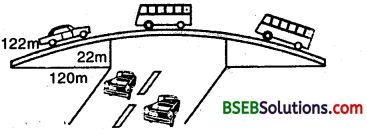Solution:
First we find the area of triangular side measuring 122 m, 22 m and 120 m.
Let a = 122 m, b = 22 m and c = 120 m.
∴ s = 12(a + b + c) = 12(122 + 22 + 120) m
= ( 12 x 264) m = 132 m
Now,
s – a = (132 – 122) m = 10 m
s – b = (132 – 22) m = 110 m
and
s – c = (132 – 120) m = 12 m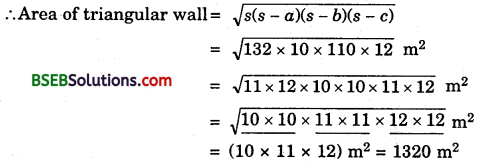Rent charges = Rs 5000 per m² per year.
∴ Rent charged from a company for 3 months
= Rs (5000 x 1320 x 312)
= Rs, 16,50,000

Question 3.
There is a slide in a park. One of its side walls has been painted in blue colour with a message “KEEP THE PARK GREEN AND CLEAN”, (See figure). If the sides of the wall are 15 m, 11 m and 6 m, find the area painted in colour.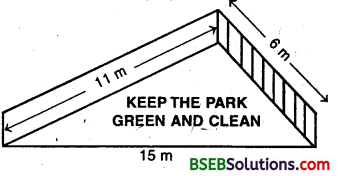Solution:
First find the area of side wall measuring 15 m, 11 m and 6 m.
Let a = 15 m, b = 11 m, c = 6 m
and s = 12 (a + b + c) = 12 (15 + 11 + 6) m
= (12 x 32) m = 16 m
Now,
s – a = (16 – 15) m = 1 m
s – b = (16 – 11) m = 5 m
and
s – c = (16 – 6) m = 10 m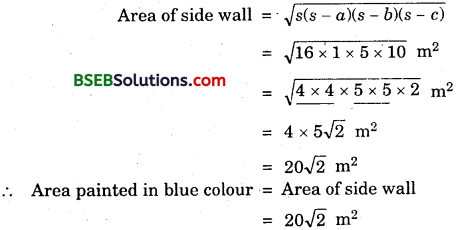Question 4.
Find the area of a triangle two sides of which are 18 cm and 10 cm and the perimeter is 42 cm.
Solution:
Let a, b and c be the sides of a triangle such that
a = 18 cm, b = 10 cm and a + b + c = 42 cm.
∴ c = 42 – a – b
⇒ c = (42 – 18 – 10) cm = 14 cm
Now, s = 12(a + b + c) = 12 x 42 cm = 21 cm
s – a = (21 – 18) cm = 3 cm
s – b = (21 – 10) cm = 11 cm
and s – c = (21 – 14) cm = 7 cmQuestion 5.
Sides of a triangle are in the ratio of 12 : 17 : 25 and its perimeter is 540 cm. Find its area.
Solution:
Let the sides be a, b and c of the triangle.
∴ a : b : c = 12 : 17 : 25 a b c
⇒ 𝑎12 = 𝑏12 = 𝑐25 = k(Say)
⇒ a = 12k, b = 17k and c = 25k
Also, perimeter = 540 cm
⇒ a + b + c = 540
⇒ 12k + 17k + 25k = 540
⇒ 54k = 540 i.e., k = 10
Thus, a = 12 x 10 = 120 cm, b = 17 x 10 cm = 170 and c = 25 x 10 = 250 cm.
s = 12 x 540 cm = 270 cm
s – a = (270 – 120) cm = 150 cm
s-b = (270 – 170) cm = 100 cm
and
s – c = (270 – 250) cm = 20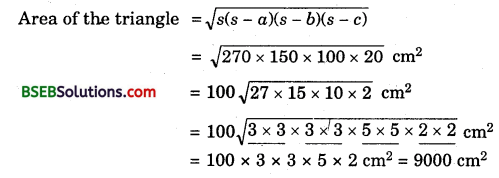Question 6.
An isosceles triangle has perimeter 30 cm and each of the equal sides is 12 cm. Find the area of the triangle.
Solution:
Here a = b = 12 cmand a + b + c = perimeter = 30 cm
⇒ c = (30 – a – b) cm
⇒ c = (30 – 12 – 12) cm = 6 cm
Now, s = 12 x 30 cm = 15 cm
s – a = (15 – 12) cm = 3 cm,
s – b = (15 – 12) cm = 3 cm
and
s – c = (15 – 6) cm = 9 cm## Bihar Board Class 9th Maths Chapter 12 Heron’s Formula Ex 12.1 Textbooks for Exam Preparations

Bihar Board Class 9th Maths Chapter 12 Heron’s Formula Ex 12.1 Textbook Solutions can be of great help in your Bihar Board Class 9th Maths Chapter 12 Heron’s Formula Ex 12.1 exam preparation. The BSEB STD 9th Maths Chapter 12 Heron’s Formula Ex 12.1 Textbooks study material, used with the English medium textbooks, can help you complete the entire Class 9th Maths Chapter 12 Heron’s Formula Ex 12.1 Books State Board syllabus with maximum efficiency.

## FAQs Regarding Bihar Board Class 9th Maths Chapter 12 Heron’s Formula Ex 12.1 Textbook Solutions

#### Can we get a Bihar Board Book PDF for all Classes?

Yes you can get Bihar Board Text Book PDF for all classes using the links provided in the above article.

## Important Terms

Bihar Board Class 9th Maths Chapter 12 Heron’s Formula Ex 12.1, BSEB Class 9th Maths Chapter 12 Heron’s Formula Ex 12.1 Textbooks, Bihar Board Class 9th Maths Chapter 12 Heron’s Formula Ex 12.1, Bihar Board Class 9th Maths Chapter 12 Heron’s Formula Ex 12.1 Textbook solutions, BSEB Class 9th Maths Chapter 12 Heron’s Formula Ex 12.1 Textbooks Solutions, Bihar Board STD 9th Maths Chapter 12 Heron’s Formula Ex 12.1, BSEB STD 9th Maths Chapter 12 Heron’s Formula Ex 12.1 Textbooks, Bihar Board STD 9th Maths Chapter 12 Heron’s Formula Ex 12.1, Bihar Board STD 9th Maths Chapter 12 Heron’s Formula Ex 12.1 Textbook solutions, BSEB STD 9th Maths Chapter 12 Heron’s Formula Ex 12.1 Textbooks Solutions,
Share: